Courses
Courses for Kids
Free study material
Free LIVE classes
More

# Cost Price Formula - Learning Formulas with FunLIVE
Join Vedantu’s FREE Mastercalss

## Introduction to Cost Price Formula

The cost price formula, which is also known as CP, is used to determine an item's actual price. In other words, it is the cost of any commodity that we buy. Through the selling price, the cost price aids in determining profitability.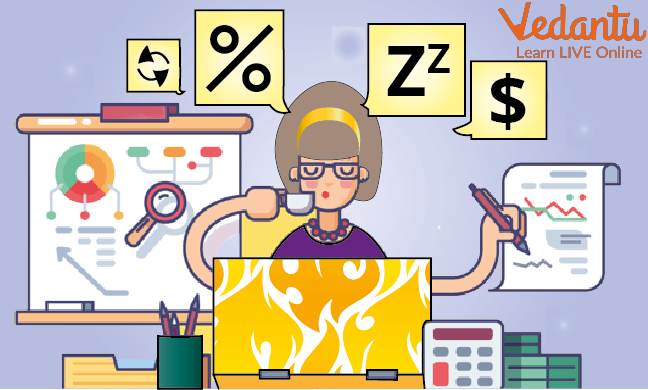Cost Price Formula

As a result, you make a profit if the original value is less than the selling price, and you lose money if the original value is higher than the selling price. In order to better understand this, we will discuss two cost price formulas in this section, understand the variables at play, and work through a few examples.

## Definition of Cost Price:The cost price is the sum of money used to produce goods or services before any profit is added for the manufacturer or producer. Other names for it include last cost, average cost, and actual cost.

The extra costs associated with production, real estate, materials, electricity, R&D, testing, worker wages, and other expenses are all included in the cost price. The cost price and the selling price of any item are always used to determine profit and loss.

## Cost Price and Selling Price: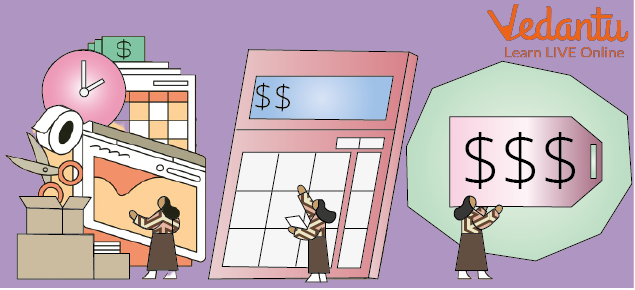Cost Price and Selling Price

• Cost Price: The cost price of an item is the sum paid to obtain it or the cost at which it was produced. The cost price is denoted by the letter C.P.

• Selling Price: The selling price is the cost at which an item is offered for sale. The selling price is denoted by the letter S.P.

## How Do You Evaluate Cost Price?Evaluating Cost Price

By comparing the profit to the selling price, the CP is used to confirm the profit. This implies that there is a profit in the purchase if the original value, which is the C.P., is lower than the selling price. Additionally, the purchase will result in a loss if the C.P. is higher than the S.P.

## What is the Cost Price Formula?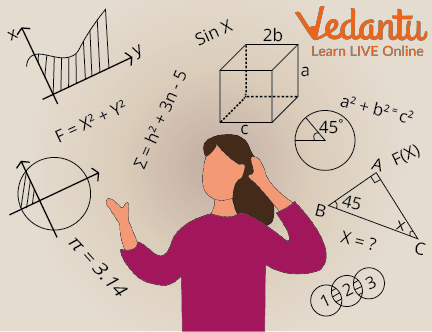Formulas

Let’s learn the cost price formula in math from the following-

• To determine whether we made money when we sold a product, we used the formula below.

The Formula for Cost Price (CP) : Selling Price (SP) - Profit

• We use the following formula if we lose money while selling a product.

The Formula for Cost Price (CP) : Selling Price (SP) + Loss

• The following is the formula for profit (gain) percentage and selling price

The Formula for Cost Price(CP) : {100/(100 + Profit %)} x SP.

• The equation based on loss percentage and SP is as follows

The Formula for Cost Price(CP) : {100/(100 - Loss %)} x SP

## Solved Examples: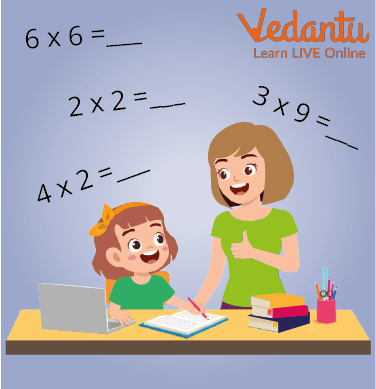Learning From Examples

1. On selling a chair for Rs500, James loses 6%. For how much did she purchase it? Calculate the cost price by using the formula.

Ans: Given, Loss = 6%; SP = Rs 500; CP = ?

If the loss is 6%, it means that if the cost price is Rs100, the loss incurred is Rs6.

If CP is Rs. 100, then SP is  Rs. 94

When SP is Rs. 94, CP = Rs. 100

When SP is Rs. 500

CP = (100/94) × 500 =  Rs531.90

∴ CP = Rs. 531.90

Therefore, the cost price of the chair is Rs. 531.90

2. If by selling a particular test series at Rs. 300 a website earns a profit of Rs. 46. then determine the CP of the test series.

Ans: Here is the selling price of the test series=Rs. 330 and profit = Rs. 46

Using the formula, we get:

CP = Selling Price - Profit

= 300-46

CP of the test series = Rs.254

## Let’s Practise: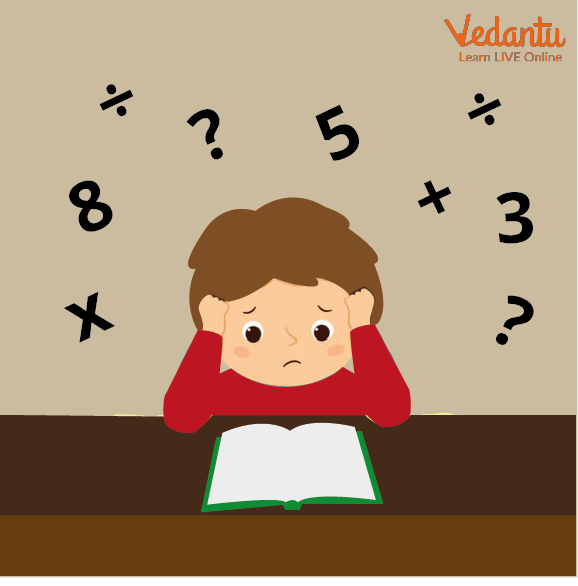Practice Time

• The selling price of a toy is Rs. 340 and the profit earned by the shopkeeper is Rs. 60. Find the cost price of the toy using the cost price formula.

Ans: Rs. 280

• An article was sold for Rs. 230 at a loss of Rs. 20. Using the cost price formula can you calculate what was its cost price?

Ans: Rs. 250

## Summary

The original cost of making a product, along with some additional costs incurred during the manufacturing process, is the cost price of the product. Calculating the actual profit of the product, its manufacturer, and its developer is helped by differences between the selling price and cost price of the products.

Last updated date: 23rd Sep 2023
Total views: 123.9k
Views today: 1.23k

## FAQs on Cost Price Formula - Learning Formulas with Fun

1. What are the selling price and cost price and how are profit and loss determined?

• Cost Price: The cost price of an item is the sum paid to acquire it or the cost at which it was produced. The cost price is denoted by the letter C.P.

• Selling Price: The selling price is the cost at which an item is offered for sale. The selling price is denoted by the letter S.P.

You must first total up all of your monthly income before calculating the profit and loss. Then total up your monthly expenditures. Subtract the total expenses from the income to determine the difference. The profit or loss is your final outcome.

2. What is the formula of total cost and what is the formula of cost price?

The variable and fixed costs of providing goods are combined to create a total using the total cost formula. The main benefit of using the total cost formula is that it provides a straightforward metric that can be measured and tracked to determine a company's profitability.

The equation is Total cost = (Average fixed cost and average variable cost) x Number of units produced.

Two different formulas are needed to calculate the loss or gain of the products and those formulas are;

• Profit = “(100*selling price or SP/ 100 + Gain %)” and;
• Loss = “(100 * SP or selling price/ 100 – Loss %)”.